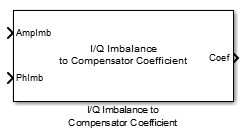# I/Q Imbalance to Compensator Coefficient

Converts amplitude and phase imbalance into I/Q compensator coefficient

## Library

RF Impairments Correction

•## Description

The I/Q Imbalance to Compensator Coefficient block returns a complex coefficient to compensate for amplitude and phase imbalance.

This block has an amplitude imbalance input port and a phase imbalance input port, where the amplitude imbalance is a real number expressed in dB and the phase imbalance is a real number expressed in degrees. The imbalance inputs are vectors. The complex coefficients are returned from a single output port.

## Algorithms

See `iqimbal2coef` for more information on the inputs, outputs, and algorithms.

## Supported Data Types

PortSupported Data Types
Compensator Coefficient
• Double-precision, complex floating point

• Single-precision, complex floating point

Amplitude Imbalance (dB)
• Double-precision floating point

• Single-precision floating point

Phase Imbalance (deg)
• Double-precision floating point

• Single-precision floating point

## Version History

Introduced in R2014b